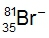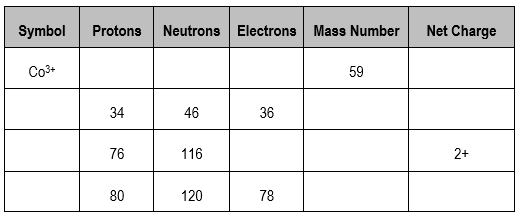Start typing, then use the up and down arrows to select an option from the list.# GOB Chemistry

Learn the toughest concepts covered in your GOB - General, Organic, and Biological Chemistry class with step-by-step video tutorials and practice problems.

Atoms and the Periodic Table

# Ions (Simplified)

Ions represent elements that possess either a positive charge or a negative charge.

## Ions and Stable Electron Arrangements

1

#### concept

Ions (Simplified) Concept 153s
Play a video:
ions are created from losing or gaining of electrons by elements in order to become like the noble gases. Now we're going to say that a metal tends to lose electrons to become a positively charged ion called a cat eye. On and non metals tend to gain electrons to become a negatively charged ion called an anti on again, elements do this in order to obtain a stable electron arrangement, like the noble gases. So that's the real reason behind them gaining or losing electrons. Now, what we need to realize is that associated with this losing and gaining electrons, we have the term isil electronic ice. Electronic just means elements have the same number of electrons. All right, So just keep in mind when we're forming ions or either gaining electrons or losing electrons.

A positively charged ion is called a cation and a negatively charged ion is called an anion.

2

#### concept

Ions (Simplified) Concept 21m
Play a video:
So when thinking of ion formation, let's take a look at boron and oxygen. All right. So for cat ion formation. Remember a cat eye on this form from the losing of electrons here, we're looking at boron 11. 11. Remember it represents its mass number or atomic mass. That's the number of protons and neutrons together. Five just gives us the number of protons within the nucleus. And if the structure is neutral, it also has five electrons, we can see the five electrons they would have. Now The five electrons are orbiting the nucleus. All of a sudden though I decided to remove three of those electrons, it now only has two electrons remaining. It just lost three electrons. So as a result born now gets a charge of three plus. Remember for each electron lost, we become more positive by one. On the other side we have our anti on formation and I'll means we're gaining electrons here. We're dealing with oxygen. 16. 16 is the mass number. Again, that's the number of protons and neutrons together. So those are found within the nucleus. Were more concerned with. Is that its atomic number is eight. Which means it has eight electrons when it's neutral. So here we see, 1, 2, 3, 4, 5, 6, 7 and eight. All of a sudden though I decided to add two additional electrons. So here we've added these two additional electrons. So it has its original eight, of course. But then I've added two more. Yeah, when you gain electrons, you gain a negative charge because I gained two electrons, oxygen's new charges now to minus. So here this is what we need to think of. When we talk about cat ion and ion formation, are we losing electrons or are we gaining electro.

Ions are formed from either the loss or gain of electrons by a neutral element.

3

#### example

Ions (Simplified) Example 12m
Play a video:
So here in this example question, it states determine the number of protons, neutrons and electrons for the following cat ion. Now it's a cat ion because it possesses a positive charge, that positive charge being three plus now, when looking at the other numbers, we know that this 13 here represents our atomic number, which uses the variables. E remember the atomic number gives us the number of protons, and because it's 13, that means we have 13 protons. That means that option. He cannot be an answer, because here we do not have 27 protons. Next, we have the number above the 13 and 27. Remember, that is your mass number, which uses the variable A. Your mass number gives you the number of protons plus neutrons within the element or ion. From this information, we know that the number off neutrons would equal a minus Z, so it's the mass number minus the atomic number, So that would be 27 minus 13, which would give us 14 neutrons. So so far, options B and C can be the only correct choices because in a we do not have 27 new trials. Now the charge is three plus three plus means that you have lost electrons. It means you have lost three electrons. When aluminum is neutral has no charge. It has an equal number of protons and electrons. Okay, but now we've lost three electrons. So what does that mean? That means we have 10 electrons remaining. So the answer would have to be see here. And if you want to double check that, you could just say we have 13 protons and 10 electrons, so we know that would be plus 13 plus minus 10, which would give us plus three as an answer. That proves that our charge should be plus three here. So, out of all the choices present Onley option C gives the correct number for each of the subatomic particles.
4
Problem

Give the correct number of protons, neutrons and electrons for the following isotope:.

5
Problem

In which pair are the two species both isoelectronic and isotopic?

6
Problem

One isotope of a metallic element has a mass number of 65 and 35 neutrons in the nucleus. The cation that this atom forms has 28 electrons. What is the symbol of the cation?

7
Problem

Which of the following is the symbol for the ion with a +4 charge, 30 neutrons and 21 electrons?

8
Problem

Fill in the gaps for the following table.